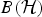Hostname: page-component-5db6c4db9b-mcx2m Total loading time: 0 Render date: 2023-03-24T17:32:08.190Z Has data issue: true Feature Flags: { "useRatesEcommerce": false } hasContentIssue true

# A New Class of Maximal Triangular Algebras

Published online by Cambridge University Press:  03 May 2018

## Abstract

Triangular algebras, and maximal triangular algebras in particular, have been objects of interest for over 50 years. Rich families of examples have been studied in the context of many w*- and C*-algebras, but there remains a dearth of concrete examples in$B({\cal H})$. In previous work, we described a family of maximal triangular algebras of finite multiplicity. Here, we investigate a related family of maximal triangular algebras with infinite multiplicity, and unearth a new asymptotic structure exhibited by these algebras.

## MSC classification

Type
Research Article
Information
Proceedings of the Edinburgh Mathematical Society , November 2018 , pp. 909 - 931

## Access options

Get access to the full version of this content by using one of the access options below. (Log in options will check for institutional or personal access. Content may require purchase if you do not have access.)

## References

1Davidson, K. R., Similarity and compact perturbations of nest algebras, J. Reine Angew. Math. 348 (1984), 286294.Google Scholar
2Davidson, K. R., Nest algebras (eds Brezis, H., Douglas, R. G. and Jeffrey, A.), Research Notes in Mathematics, Volume 191, pp. 1412 (Pitman, Boston, MA, 1988).Google Scholar
3Erdos, J. A., Unitary invariants for nests, Pacific J. Math. 23 (1967), 229256.CrossRefGoogle Scholar
4Kadison, R. V., Triangular algebras–another chapter, In Selfadjoint and nonselfadjoint operator algebras and operator theory (ed. Doran, R. S.), Contemporary Mathematics, Volume 120, pp. 6376 (CBMS), (American Mathematical Society, 1991).CrossRefGoogle Scholar
5Kadison, R. V. and Singer, I. M., Extensions of pure states, Amer. J. Math. 81 (1959), 383400.CrossRefGoogle Scholar
6Kadison, R. V. and Singer, I. M., Triangular operator algebras, Amer. J. Math. 82(2) (1960), 227259.CrossRefGoogle Scholar
7Larson, D. R., Nest algebras and similarity transformations, Ann. Math. 121 (1985), 409427.CrossRefGoogle Scholar
8Muhly, P. S., Saito, K.-S. and Solel, B., Coordinates for triangular operator algebras, Ann. of Math. (2) 127 (1988), 245278.CrossRefGoogle Scholar
9Muhly, P. S. and Solel, B., Subalgebras of groupoid C*-algebras, J. Reine Angew. Math. 402 (1989), 4175.Google Scholar
10Orr, J. L., On the closure of triangular algebras, Amer. J. Math. 112 (1990), 481497.CrossRefGoogle Scholar
11Orr, J. L., An estimate on the norm of the product of infinite block operator matrices, J. Combin. Theory Ser. A 63(2) (1993), 195209.Google Scholar
12Orr, J. L., The maximal ideals of a nest algebra, J. Func. Anal. 124 (1994), 119134.CrossRefGoogle Scholar
13Orr, J. L., Triangular algebras and ideals of nest algebras, Mem. Amer. Math. Soc. 117(562) 1995, 49pp.Google Scholar
14Orr, J. L., The stable ideals of a continuous nest algebra, J. Operator Theory 45 (2001), 377412.Google Scholar
15Peters, J. R., Poon, Y. T. and Wagner, B. H., Triangular AF algebras, J. Operator Theory 23(1) (1990), 81114.Google Scholar
16Poon, Y. T., Maximal triangular subalgebras need not be closed, Proc. Amer. Math. Soc. (1991), 475479.CrossRefGoogle Scholar
17Power, S. C., The classification of triangular subalgebras of AF C*-algebras, Bull. Lond. Math. Soc. 22(3) (1990), 269272.CrossRefGoogle Scholar
18Ringrose, J. R., On some algebras of operators, Proc. London Math. Soc. 15(3) (1965), 6183.CrossRefGoogle Scholar
19Solel, B., Irreducible triangular algebras, Volume 290 (American Mathematical Society, 1984).Google Scholar